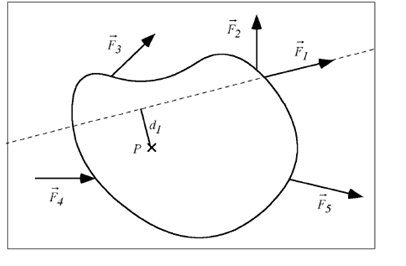# Problem: Now we draw the line of action for each force. This is the line along which the force acts, and it extends in both directions. The line of action for force F1 is shown here as a dashed line. Draw the line of action for the other four forces in the diagram.Next we find the shortest distance. This is the perpendicular distance from the line of action to the pivot point, and is shown for force F1 labeled as d1. You should show this distance for the other four forces

###### FREE Expert Solution

The line of action of F5 passes through point P.

Therefore, f F5, d5 = 0

80% (207 ratings)###### Problem Details

Now we draw the line of action for each force. This is the line along which the force acts, and it extends in both directions. The line of action for force F1 is shown here as a dashed line. Draw the line of action for the other four forces in the diagram.Next we find the shortest distance. This is the perpendicular distance from the line of action to the pivot point, and is shown for force F1 labeled as d1. You should show this distance for the other four forces